REGRESSION ANALYSIS BASICS
Different regression techniques give different results for the regression equation. Simple or Linear regression is the most common form used in petrophysical analysis, giving an equation of the form Y = A * X + B.

Multiple regression related the dependent variable Y to a number of independent variables, for example Y = A1 * X1 + A2 * X2 ... +B.

Non linear or polynomial regression provides relationships that involve powers, roots, or other non-linear functions, such as logarithms or exponentials.

Excel and Lotus 1-2-3 offer some simple linear and non-linear regression models, but more sophisticated software is required for multiple regression. A good freeware package is Statcato (www.statcato.org). It is a java based program: right-click and "Save Target As" >> Stats / Regression Package, unzip the files to a folder, and click "Statcato.jar".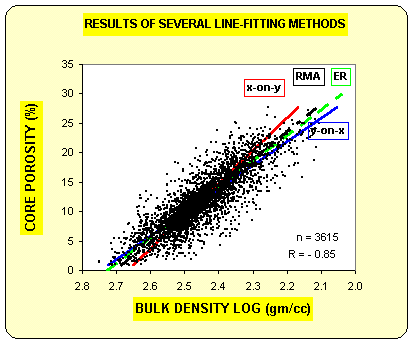The graph at left (courtesy Dick Woodhouse) shows four different lines. The "Y-on-X" line is the one that will result from use of spreadsheet software. Y is the dependent axis (predicted variable) and X is the independent axis (the variable doing the predicting). The line minimized the errors in the vertical direction (Y axis) using a least-squares solution.

The "X-on-Y line reverses the roles of the two axes, minimizing the error in the horizontal direction (as the graph is drawn here)..

The RMA line, the reduced major axis, assumes that neither axis depends on the other and is very nearly halfway between the first two lines. It minimizes the error at right angles to the line. The ER, or error ratio line, minimizes the error on both X and Y directions. There is not usually much difference between the RMA and ER lines. All four lines intersect at the centroid of the data.SIMPLE LINEAR REGRESSION and BASIC Statistical
The equations used are as follows:Slope of Best Fit Line
1: A1 = (Sum (XiYi) - Sum (Xi) * Sum (Yi) / Ns) / (Sum (Xi ^ 2) - Sum (Xi) ^ 2) / Ns)
2: A2 = (Sum (XiYi) - Sum (Yi) * Sum (Xi) / Ns) / (Sum (Yi ^ 2) - Sum (Yi) ^ 2) / Ns)Intercept on Y Axis
3: B1 = (Sum (Yi) - Al * Sum (Xi)) / Ns
4: B2 = (Sum (Xi) - A2 * Sum (Yi)) / Ns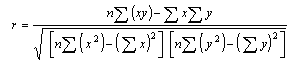Equation of Best Fit Lines
5: Y = A1 * X + B1  (Y is dependent axis)
6: X = A2 * Y + B2 (X is dependent axis)

The Reduced Major Axis regression line is the regression line that usually represents the most useful relationship between the X and Y axes. It assumes that both axes are equally error prone. An approximation to this line is halfway between the two independent regression lines. Solve equation 6 for Y:
7: Y = (1/A2) * X + B2 / A2

Average slope and intercept of equations 5 and 7:
8: A3 = (A1 + 1/A2) / 2
9: B3 = (B1 + B2 / A2) / 2
10: Y = A3 * X + B3 (reduced major axis)

Coefficient of Determination
11: Cd = (B1 * Sum (iY) + Al * Sum (Xi * Yi) - (Sum (Yi) ^ 2) / Ns) /
(Sum (Xi ^ 2) - (Sum (Xi) ^ 2) / Ns)

The coefficient of determination is a measure of "best fit" and is capable of being calculated as data is entered and processed (e.g.: as in a hand calculator). Other measures of fit require two passes through the data - the first to find the average X and average Y values, then a second pass to find the differences between each individual X and the average X, and the differences between the individual Y and the average Y values.

An alternate form of the above equation is:
12: Cd = (Sum (XiYi) - Sum (Xi) * Sum (Yi) / Ns) / (((Sum (Xi ^ 2) - Sum (Xi) ^ 2) / Ns) *
(Sum (Yi ^ 2) - Sum (Yi) ^ 2) / Ns)) ^ 0.5

Both equations give the same answer.

These data are used in the following statistical measures.

Arithmetic Mean
13: Xbar = Sum (Xi) / Ns
14: Ybar = Sum (Yi) / Ns

Variance
15: Vx = Sum ((Xi - Xbar) ^ 2) / (Ns - 1)
16: Vy = Sum ((Yi - Ybar) ^ 2) / (Ns - 1)

Standard Deviation
17: Sx = Vx ^ 0.5
18: Sy = Vy ^ 0.5

Correlation Coefficient
19: Rxy = A1 * Sx / Sy

T Ratio
20: Txy = Rxy * ((Ns - 2) / (1 - (Rxy ^ 2))) ^ 0.5

Skew
21: Ux = (Sum ((Xi - Xbar) ^ 3) / Ns) / ((Sum ((Xi - Xbar) ^ 2) / Ns) ^ 1.5)
22: Uy = (Sum ((Yi - Ybar) ^ 3) / Ns) / ((Sum ((Yi - Ybar) ^ 2) / Ns) ^ 1.5)

Kurtosis
23: Kx = (Sum ((Xi - Xbar) ^ 4) / Ns) / ((Sum ((Xi - Xbar) ^ 2) / Ns) ^ 2)
24: Ky = (Sum ((Yi -Ybar) ^ 4) / Ns) / ((Sum ((Yi - Ybar) ^ 2) / Ns) ^ 2)

Geometric Mean
25: Gx = (PROD (Xi)) ^ (1 / Ns)
26: Gy = (PROD (Yi)) ^ (1 / Ns)

Harmonic Mean
27: Hx = Ns / (Sum (1 / Xi))
28: Hy = Ns / (Sum (1 / Yi))

Where:
A1 = slope of best fit line (x dependent)
A2 = slope of best fit line (y dependent)
A3 = slope of best fit line (reduced major axis)
B1 = intercept of best fit line (x dependent)
B2 = intercept of best fit line (y dependent)
B3 = intercept of best fit line (reduced major axis)
Cd = coefficient of determinations
Gx = geometric mean of X values
Gy = geometric mean of Y values
Hx = harmonic mean of X values
Hy = harmonic mean of Y values
Kx = kurtosis of X values
Ky = kurtosis of Y values
Ns = number of X - Y pairs or number of samples
Rxy = correlation coefficient
Sx = standard deviation of X values
Sy = standard deviation of Y values
Txy = T ratio
Ux = skew of X values
Uy = skew of Y values
Vx = variance of X values
Vy = variance of Y values
Xi = individual X data values
Xbar = arithmetic mean of X values
XiYi = product of individual X - Y pairs
Yi = individual Y data values
Ybar = arithmetic mean of Y valuesMULTIPLE  LINEAR REGRESSION
The model for a multiple regression takes the form:
30:
Y = b0 + b1X1 + b2X2 + b3X3 + .....

The b's are termed the "regression coefficients".  Instead of fitting a line to data, we are now fitting a plane (for 2 independent variables), a space (for 3 independent variables).

The estimation can still be done according the principles of linear least squares. The algebraic formulae for the solution (i.e. finding all the b's) are UGLY. However, the matrix solution is elegant:

The matrix model is:
31:  [Y] = [X] * [B]

The solution is:
32: [B] = ([X'] * [X])-1 * [X'] * [Y]CROSSPLOTS
Crossplots assist in selection of petrophysical parameters, identification of trends and problems, and compress large amounts of data into a small space. Several hundred thousand different crossplots could be made on the same zone, but only a few are helpful. Some of these are described in detail here. Most of the crossplots on this page also show up in appropriate sections elsewhere in this Handbook, close to the topic that makes use of the data.

Statistical analysis of data, such as regression analysis or frequency distributions, can be described both graphically and mathematically. The math for very basic statistical analysis of petrophysical data is covered here.

The majority of crossplots are X - Y coordinate graphs, often called scatter plots. They are useful for showing the relationship between two measurements, for example, resistivity versus gamma ray readings. By making the symbol that is plotted vary in colour with a third parameter, for example the PE curve, we have a 3-D crossplot. In this case it shows the variation of lithology with changes in resistivity and gamma ray value.

Although not widely used, the shape of the characters used to plot each data point can be varied to represent a fourth variable, for example the frequency of occurrence of data at this location on the plot. These are 4-D plots, invented by the author in 1976.

Groupings of data may represent important petrophysical parameters, such as shale properties, water or hydrocarbon zone location, or mineralogy. The use of a particular crossplot is dictated by common sense rules. Some crossplots, especially those related to mineralogy, benefit from a background template showing the location of the pure mineral values observed in the laboratory.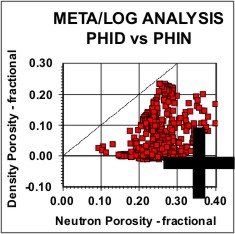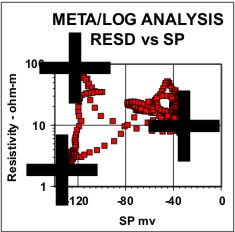Crossplots used to locate density and neutron shale points (left), gamma ray clean and shale points (middle) and SP clean and shale points (right). Heavy crosses indicate outer boundaries of the chosen data. Shale resistivity, water zone resistivity, and maximum resistivity in clean sand can also be picked on the GR and SP plots.Histograms of the distribution of log data are used for choosing petrophysical properties, as in the GR example at left. They are also used to help in normalizing log data between wells by suggesting the linear shift needed to match the distribution from a model or key well.

Regression analysis of log data, or core versus log data, is very commonly used to find relationships that predict or calibrate petrophysical results, as at the right. The equation of the best fit line can be used in user-defined equation sets in most computer or spreadsheet software.The typical use for crossplots of core data is to determine the equation relating permeability to porosity, as shown at the left. Even though the equation can always be derived, the regression line will not useful if the data spread is too large,

The other common crossplot with core data are regressions of core porosity against sonic, density, neutron, or answer porosity, used to establish calibration equations.CROSSPLOT EXAMPLES - Shaly Sand

The raw logs show two zones of interest: a lower clean sand with hydrocarbon over water and a very poor quality upper shaly zone with a hydrocarbon indication. These zones can be spotted by laying the density log over the resistivity log and looking for the crossover of the curves. Because the sands are not pure quartz, a conventional shaly sand analysis technique is not appropriate because it would underestimate porosity, so a complex lithology model was used instead.

There is no density neutron crossover in the clean sand, so this zone is oil bearing. We cannot tell about the upper shaly sand because the shale effect masks any possible gas effect. After shale corrections, the density and neutron still do not cross over, so oil is most likely.

The water zone at the base of the clean sand provides water resistivity information for use throughout the rest of the zone. Core data was available to calibrate porosity and permeability results. The answer plot shows the results of the lithology, porosity, and hydrocarbon analysis.

The raw data plot shows two interesting features: the flat SP compared to GR in tight zones and the SP excess at 3400 feet, indicating better permeability than the rest of the shaly sand. The lithology track on the answer plot shows this interval to be more sandy and less limey than the rest of the shaly sand.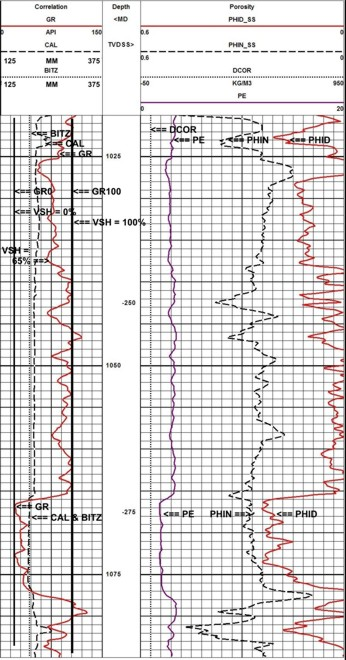Raw logs for Shaly Sand Example

1.       Porosity vs Resistivity - shows water saturation lines (shale data falls below 100% Sw line).

2.       Porosity vs Saturation - shows constant water volume lines. Data streaming above and to the right indicate transition and water zones. Shale data falls to the bottom of the graph.

3.       Density vs Neutron - shows all data below limestone line, indicating either no perfectly clean sand or mixed lithology sand (GR suggests clean sand). Shale data falls towards bottom and right.

4.       Core porosity vs core permeability - shows a data cluster which cannot be used to derive a regression line mathematically. A line drawn thru the lower left corner will work fine.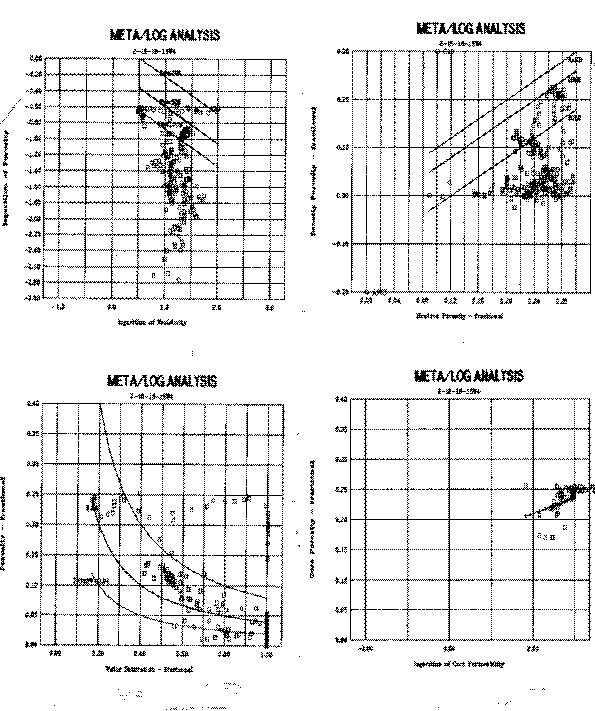Basic crossplots for Shaly Sand Example  - Part 1

5.       Matrix density vs matrix cross section - confirms that sand is not pure quartz, but the plot does not tell us which minerals to expect. Sample description suggests quartz, calcite, and glauconite (plots past anhydrite at top right).

Apparent water resistivity vs density - shows RW@FT and RWSH points relative to spread of data for both shale and hydrocarbon zones.

7.      Apparent water resistivity vs density porosity - similar to above but uses effective porosity. Shale plots near origin, water zone at top left, oil at right.

8.       Apparent water resistivity vs gamma ray - shows where to pick GR0 and GR100 (also can be picked from raw logs). Best oil zone is off scale to the right.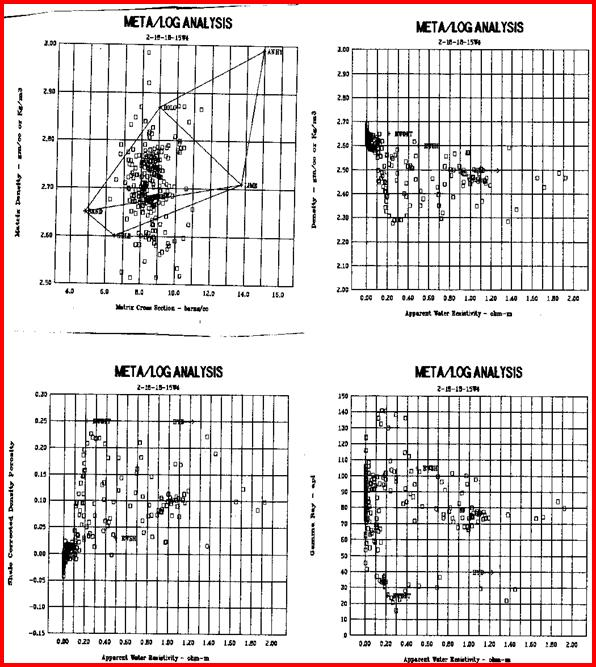Basic crossplots for Shaly Sand Example  - Part 2Cumulative (Holgate) Plots
A Holgate plot is a special crossplot constructed in order to calibrate one log response to another, or to calibrate a log response or computed result with core data. The usual form is a sonic log versus core porosity plot, but any two co relatable properties may be compared. However, the construction is quite a bit more complicated than merely plotting X-Y data as in previous plots. A Holgate plot requires cumulative data over an interval of the formation. For example, assume a series of log or core values such as:

 Sample #: 1 2 3 4 5 6 7 8 9 Data Value: 0 2 4 6 8 6 4 2 0

The data is sorted into ascending (or descending) values and placed into cells with discrete ranges:

 Data Values Represented (Range) 0-1.9 2-3.9 4-5.9 6-7.9 8-9.9 Number of Samples in Each Range 2 2 2 2 1 Number of Samples Accumulated 2 4 6 8 9

The crossplot is created by plotting the lower row of numbers (the accumulated number of samples) on the Y axis versus the centroid of the range of data values represented on the X axis. Usually these points are connected by a series of straight lines. If the range of values in each cell is very small, a smooth cumulative curve can be created. This is normally done on a computer.

If two such curves are made, one for a log value, and the other for a core property such as porosity, a calibration curve can be constructed. Assume our previous data reflected core porosity data and the sonic data had the following values:

 Data Range 50-54 55-59 60-64 65-69 70-75 Number of Samples 1 2 2 2 2 Accumulation 1 3 5 7 9

The resulting calibration would relate the centroid of each range to its corresponding value in the other table. Thus:

 Core Porosity 1 3 5 7 9 Sonic Log Reading 52.5 57.5 62.5 67.5 72.5

A best fit regression analysis on this paired data would generate the equation of the line which calibrates sonic log readings to porosity. The relationship need not be linear.

The data for the two sets of values must come from the same interval of rock, but the two sets do not need to be "on depth" with each other since no actual depth values are used. In fact, an upside-down core will still produce the same log calibration as a right-side-up core.

Although the Y-axis accumulations were a number of samples in this example, the accumulation can be any one of:
- frequency of occurrence (same as number of samples)
- actual thickness
- percent or fractional frequency
- percent or fractional thickness

A compact form of this plot comprises three separate plots on one page, with axes appropriately labeled. The three plots are:
1. Number of samples versus data-type-one accumulated in ascending order
2. Number of samples versus data-type-two accumulated in descending order
3. Values of data-type-one versus data-type-two picked from the accumulated curves at equal intervals

The first two curves will create two "S" shaped curves facing in opposite directions and crossing at their median values. The third curve, when fitted with a regression line, will provide the calibration equation.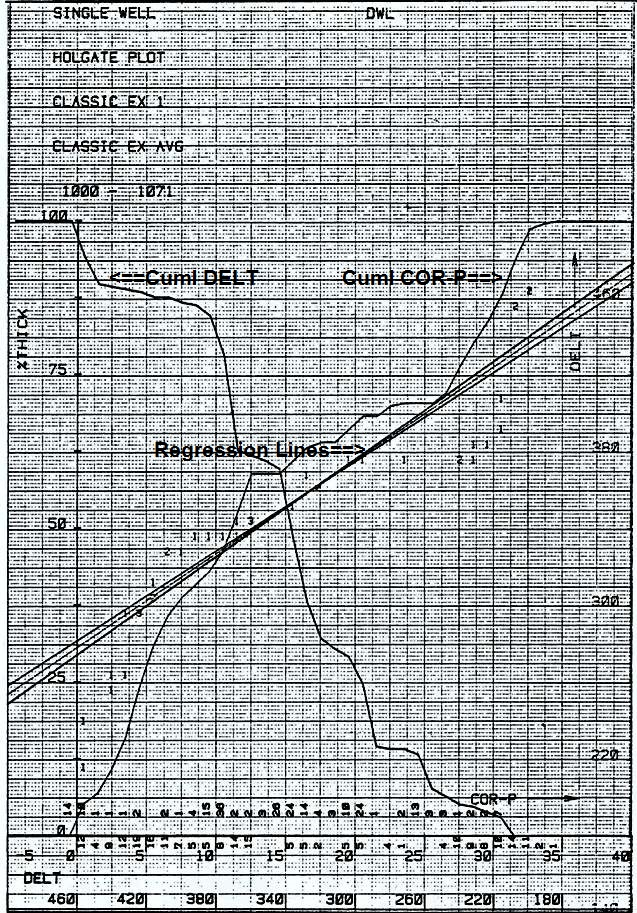This is a sonic versus core porosity Holgate plot. The S-shaped curve on the left is DELT vs cumulative percent thickness (sonic scale increases from right to left). The S-shaped curve on the right is core porosity vs cumulative percent thickness (scale increase from left to right). The three regression lines are sonic on vertical axis (scale is on right edge of plot) vs core porosity on the horizontal axis (scale is near bottom of graph above the DELT scale). Lines represent regression of X on Y, reduced major axis, and Y on X. The three lines are very close to each other, suggesting a good correlation of the two cumulative curves. The actual equations and regression coefficient are shown below.Regression results for the Holgate plot shown above.

Page Views ---- Since 01 Jan 2015
Copyright 2023 by Accessible Petrophysics Ltd.
CPH Logo, "CPH", "CPH Gold Member", "CPH Platinum Member", "Crain's Rules", "Meta/Log", "Computer-Ready-Math", "Petro/Fusion Scripts" are Trademarks of the Author